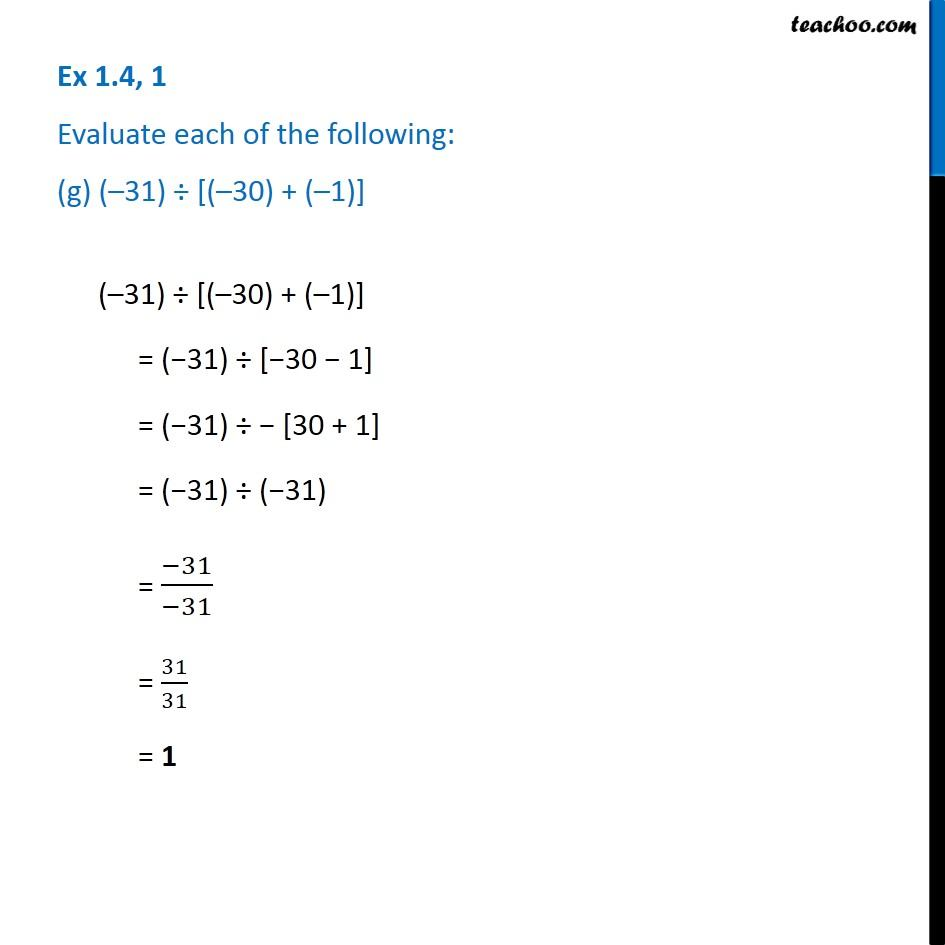Ex 1.4

Chapter 1 Class 7 Integers
Serial order wiseGet live Maths 1-on-1 Classs - Class 6 to 12

### Transcript

Ex 1.4, 1 Evaluate each of the following: (g) ( 31) [( 30) + ( 1)] ( 31) [( 30) + ( 1)] = ( 31) [ 30 1] = ( 31) [30 + 1] = ( 31) ( 31) = ( 31)/( 31) = 31/31 = 1